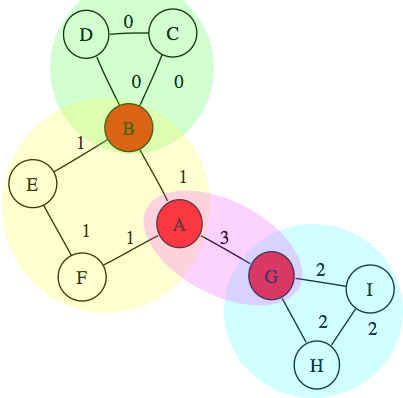#Boost C++ Libraries

...one of the most highly regarded and expertly designed C++ library projects in the world.#biconnected_components and articulation_points

```// named parameter version
template <typename Graph, typename ComponentMap, typename OutputIterator,
typename P, typename T, typename R>
std::pair<std::size_t, OutputIterator>
biconnected_components(const Graph& g, ComponentMap comp, OutputIterator out,
const bgl_named_params<P, T, R>& params)

template <typename Graph, typename ComponentMap,
typename P, typename T, typename R>
std::size_t
biconnected_components(const Graph& g, ComponentMap comp,
const bgl_named_params<P, T, R>& params)

template <typename Graph, typename OutputIterator,
typename P, typename T, typename R>
OutputIterator articulation_points(const Graph& g, OutputIterator out,
const bgl_named_params<P, T, R>& params)

// non-named parameter version
template <typename Graph, typename ComponentMap, typename OutputIterator,
typename DiscoverTimeMap, typename LowPointMap>
std::pair<std::size_t, OutputIterator>
biconnected_components(const Graph& g, ComponentMap comp, OutputIterator out,
DiscoverTimeMap discover_time, LowPointMap lowpt);

template <typename Graph, typename ComponentMap, typename OutputIterator>
std::pair<std::size_t, OutputIterator>
biconnected_components(const Graph& g, ComponentMap comp, OutputIterator out);

template <typename Graph, typename ComponentMap>
std::size_t biconnected_components(const Graph& g, ComponentMap comp);

template <typename Graph, typename OutputIterator>
OutputIterator articulation_points(const Graph& g, OutputIterator out);
```

A connected graph is biconnected if the removal of any single vertex (and all edges incident on that vertex) can not disconnect the graph. More generally, the biconnected components of a graph are the maximal subsets of vertices such that the removal of a vertex from a particular component will not disconnect the component. Unlike connected components, vertices may belong to multiple biconnected components: those vertices that belong to more than one biconnected component are called articulation points or, equivalently, cut vertices. Articulation points are vertices whose removal would increase the number of connected components in the graph. Thus, a graph without articulation points is biconnected. The following figure illustrates the articulation points and biconnected components of a small graph:Vertices can be present in multiple biconnected components, but each edge can only be contained in a single biconnected component. The output of the biconnected_components algorithm records the biconnected component number of each edge in the property map comp. Articulation points will be emitted to the (optional) output iterator argument to biconnected_components, or can be computed without the use of a biconnected component number map via articulation_points. These functions return the number of biconnected components, the articulation point output iterator, or a pair of these quantities, depending on what information was recorded.

### Parameters

IN: const Graph& g
An undirected graph. The graph type must be a model of Vertex List Graph and Incidence Graph.
Python: The parameter is named graph.
OUT: ComponentMap c
The algorithm computes how many biconnected components are in the graph, and assigning each component an integer label. The algorithm then records which component each edge in the graph belongs to by recording the component number in the component property map. The ComponentMap type must be a model of Writable Property Map. The value type shouch be an integer type, preferably the same as the edges_size_type of the graph. The key type must be the graph's edge descriptor type.
Default: dummy_property_map.
Python: Must be an edge_int_map for the graph.
Python default: graph.get_edge_int_map("bicomponent")
OUT: OutputIterator out
The algorithm writes articulation points via this output iterator and returns the resulting iterator.
Default: a dummy iterator that ignores values written to it.
Python: this parameter is not used in Python. Instead, both algorithms return a Python list containing the articulation points.

### Named Parameters

IN: vertex_index_map(VertexIndexMap i_map)
This maps each vertex to an integer in the range [0, num_vertices(g)). The type VertexIndexMap must be a model of Readable Property Map. The value type of the map must be an integer type. The vertex descriptor type of the graph needs to be usable as the key type of the map.
Default: get(vertex_index, g)
Python: Unsupported parameter.
UTIL/OUT: discover_time_map(DiscoverTimeMap discover_time)
The discovery time of each vertex in the depth-first search. The type DiscoverTimeMap must be a model of Read/Write Property Map. The value type of the map must be an integer type. The vertex descriptor type of the graph needs to be usable as the key type of the map.
Default: an iterator_property_map created from a std::vector of vertices_size_type of size num_vertices(g) and using get(vertex_index, g) for the index map.
Python: Unsupported parameter.
UTIL/OUT: lowpoint_map(LowPointMap lowpt)
The low point of each vertex in the depth-first search, which is the smallest vertex reachable from a given vertex with at most one back edge. The type LowPointMap must be a model of Read/Write Property Map. The value type of the map must be an integer type. The vertex descriptor type of the graph needs to be usable as the key type of the map.
Default: an iterator_property_map created from a std::vector of vertices_size_type of size num_vertices(g) and using get(vertex_index, g) for the index map.
Python: Unsupported parameter.
UTIL/OUT: predecessor_map(PredecessorMap p_map)
The predecessor map records the depth first search tree. The PredecessorMap type must be a Read/Write Property Map whose key and value types are the same as the vertex descriptor type of the graph.
Default: an iterator_property_map created from a std::vector of vertex_descriptor of size num_vertices(g) and using get(vertex_index, g) for the index map.
Python: Must be a vertex_vertex_map for the graph.
IN: visitor(DFSVisitor vis)
A visitor object that is invoked inside the algorithm at the event-points specified by the DFS Visitor concept. The visitor object is passed by value .
Default: dfs_visitor<null_visitor>
Python: The parameter should be an object that derives from the DFSVisitor type of the graph.

### Complexity

The time complexity for the biconnected components and articulation points algorithms O(V + E).

### Example

The file examples/biconnected_components.cpp contains an example of calculating the biconnected components and articulation points of an undirected graph.

### Notes

 Since the visitor parameter is passed by value, if your visitor contains state then any changes to the state during the algorithm will be made to a copy of the visitor object, not the visitor object passed in. Therefore you may want the visitor to hold this state by pointer or reference.

 Copyright © 2000-2004 Jeremy Siek, Indiana University (jsiek@osl.iu.edu) Doug Gregor, Indiana University